International
Tables for
Crystallography
Volume B
Reciprocal space
Edited by U. Shmueli

International Tables for Crystallography (2010). Vol. B, ch. 2.2, pp. 230-231   | 1 | 2 |

Section 2.2.6. Direct methods in real and reciprocal space: Sayre's equation

C. Giacovazzoa*

aDipartimento Geomineralogico, Campus Universitario, 70125 Bari, Italy, and Institute of Crystallography, Via G. Amendola, 122/O, 70125 Bari, Italy
Correspondence e-mail: carmelo.giacovazzo@ic.cnr.it

2.2.6. Direct methods in real and reciprocal space: Sayre's equation

| top | pdf |

The statistical treatment suggested by Wilson for scaling observed intensities corresponds, in direct space, to the origin peak of the Patterson function, so it is not surprising that a general correspondence exists between probabilistic formulation in reciprocal space and algebraic properties in direct space.

For a structure containing atoms which are fully resolved from one another, the operation of raisingto the nth power retains the condition of resolved atoms but changes the shape of each atom. Letwhereis an atomic function andis the coordinate of the `centre' of the atom. Then the Fourier transform of the electron density can be written as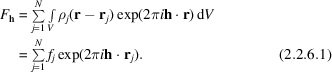If the atoms do not overlapand its Fourier transform gives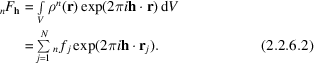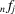is the scattering factor for the jth peak of: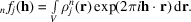We now introduce the condition that all atoms are equal, so thatand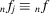for any j. From (2.2.6.1)and (2.2.6.2)we may write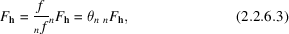whereis a function which corrects for the difference of shape of the atoms with electron distributionsand. Since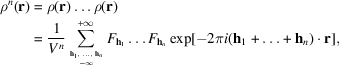the Fourier transform of both sides gives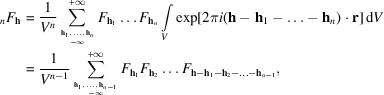from which the following relation arises: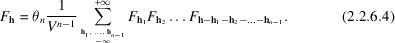For, equation (2.2.6.4)reduces to Sayre's (1952) equation [but see also Hughes (1953)]If the structure contains resolved isotropic atoms of two types, P and Q, it is impossible to find a factorsuch that the relationholds, since this would imply values ofsuch thatandsimultaneously. However, the following relationship can be stated (Woolfson, 1958):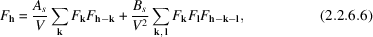whereandare adjustable parameters of. Equation (2.2.6.6)can easily be generalized to the case of structures containing resolved atoms of more than two types (von Eller, 1973).

Besides the algebraic properties of the electron density, Patterson methods also can be developed so that they provide phase indications. For example, it is possible to find the reciprocal counterpart of the functionForthe function (2.2.6.7)coincides with the usual Patterson function; for, (2.2.6.7)reduces to the double Patterson functionintroduced by Sayre (1953). Expansion ofas a Fourier series yieldsVice versa, the value of a triplet invariant may be considered as the Fourier transform of the double Patterson.

Among the main results relating direct- and reciprocal-space properties it may be remembered:

 (a) from the properties ofthe following relationship may be obtained (Vaughan, 1958)which is clearly related to (2.2.5.12); (b) the zero points in the Patterson function provide information about the value of a triplet invariant (Anzenhofer & Hoppe, 1962; Allegra, 1979); (c) the Hoppe sections (Hoppe, 1963) of the double Patterson provide useful information for determining the triplet signs (Krabbendam & Kroon, 1971; Simonov & Weissberg, 1970); (d) one phase s.s.'s of the first rank can be estimated via the Fourier transform of single Harker sections of the Patterson (Ardito et al., 1985), i.e.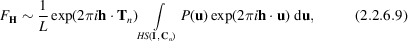where (see Section 2.2.5.9)is the s.s., u varies over the complete Harker section corresponding to the operator[in symbols] and L is a constant which takes into account the dimensionality of the Harker section. If no spurious peak is on the Harker section, then (2.2.6.9)is an exact relationship. Owing to the finiteness of experimental data and to the presence of spurious peaks, (2.2.6.9)cannot be considered in practice an exact relation: it works better when heavy atoms are in the chemical formula. More recently (Cascarano, Giacovazzo, Luić et al., 1987), a special least-squares procedure has been proposed for discriminating spurious peaks among those lying on Harker sections and for improving positional and thermal parameters of heavy atoms. (e) translation and rotation functions (see Chapter 2.3), when defined in direct space, always have their counterpart in reciprocal space.

References

Allegra, G. (1979). Derivation of three-phase invariants from the Patterson function. Acta Cryst. A35, 213–220.
Anzenhofer, K. & Hoppe, W. (1962). Phys. Verh. Mosbach. 13, 119.
Ardito, G., Cascarano, G., Giacovazzo, C. & Luić, M. (1985). 1-Phase seminvariants and Harker sections. Z. Kristallogr. 172, 25–34.
Cascarano, G., Giacovazzo, C., Luić, M., Pifferi, A. & Spagna, R. (1987). 1-Phase seminvariants and Harker sections. II. A new procedure. Z. Kristallogr. 179, 113–125.
Eller, G. von (1973). Génération de formules statistiques entre facteurs de structure: la méthode du polynome. Acta Cryst. A29, 63–67.
Hoppe, W. (1963). Phase determination and zero points in the Patterson function. Acta Cryst. 16, 1056–1057.
Hughes, E. W. (1953). The signs of products of structure factors. Acta Cryst. 6, 871.
Krabbendam, H. & Kroon, J. (1971). A relation between structure factor, triple products and a single Patterson vector, and its application to sign determination. Acta Cryst. A27, 362–367.
Sayre, D. (1952). The squaring method: a new method for phase determination. Acta Cryst. 5, 60–65.
Sayre, D. (1953). Double Patterson function. Acta Cryst. 6, 430–431.
Simonov, V. I. & Weissberg, A. M. (1970). Calculation of the signs of structure amplitudes by a binary function section of interatomic vectors. Sov. Phys. Dokl. 15, 321–323.
Vaughan, P. A. (1958). A phase-determining procedure related to the vector-coincidence method. Acta Cryst. 11, 111–115.
Woolfson, M. M. (1958). Crystal and molecular structure of p,p′-dimethoxybenzophenone by the direct probability method. Acta Cryst. 11, 277–283.